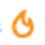$Q1$ If $S_{ n }$ is the sum

$S_{ n }=1+\frac { 1 }{ 2 } +\frac { 1 }{ 3 } +.........+\frac { 1 }{ n } (n>2),\quad prove\quad that\\ n{ (n+1) }^{ \frac { 1 }{ n } }-n<{ S }_{ n }

$Q2$ If $a_{1}$, $a_{2}$, $a_{3}$,........, $a_{n}$ are positive real numbers, prove that

$\sum _{ iNote by Satyajit Ghosh
5 years, 8 months ago

This discussion board is a place to discuss our Daily Challenges and the math and science related to those challenges. Explanations are more than just a solution — they should explain the steps and thinking strategies that you used to obtain the solution. Comments should further the discussion of math and science.

When posting on Brilliant:

• Use the emojis to react to an explanation, whether you're congratulating a job well done , or just really confused .
• Ask specific questions about the challenge or the steps in somebody's explanation. Well-posed questions can add a lot to the discussion, but posting "I don't understand!" doesn't help anyone.
• Try to contribute something new to the discussion, whether it is an extension, generalization or other idea related to the challenge.

MarkdownAppears as
*italics* or _italics_ italics
**bold** or __bold__ bold
- bulleted- list
• bulleted
• list
1. numbered2. list
1. numbered
2. list
Note: you must add a full line of space before and after lists for them to show up correctly
paragraph 1paragraph 2

paragraph 1

paragraph 2

[example link](https://brilliant.org)example link
> This is a quote
This is a quote
    # I indented these lines
# 4 spaces, and now they show
# up as a code block.

print "hello world"
# I indented these lines
# 4 spaces, and now they show
# up as a code block.

print "hello world"
MathAppears as
Remember to wrap math in $$ ... $$ or $ ... $ to ensure proper formatting.
2 \times 3 $2 \times 3$
2^{34} $2^{34}$
a_{i-1} $a_{i-1}$
\frac{2}{3} $\frac{2}{3}$
\sqrt{2} $\sqrt{2}$
\sum_{i=1}^3 $\sum_{i=1}^3$
\sin \theta $\sin \theta$
\boxed{123} $\boxed{123}$

Sort by:

2) Direct using AM GM

- 5 years, 8 months ago

Can you show?and what about 1st?

- 5 years, 8 months ago

Apply am gm on a1&a2, a1&a3,...,a1&an,a2&a3........... Add them all

- 5 years, 8 months ago

Thanks I got it

- 5 years, 8 months ago

- 5 years, 8 months ago

- 5 years, 8 months ago

Did 2nd on my own

- 5 years, 8 months ago

Did you also use AM-GM?

- 5 years, 8 months ago

Yeah!!

- 5 years, 8 months ago

And first question?

- 5 years, 8 months ago

By using $A.M-G.M$ we get

$\sum_{ω=2}^{ω}\sqrt{a_{1}a_{ω}}\leq(\frac{(n-1)a_{1}+\sum_{ω=2}^{ω}a_{ω})}{2}$

$\sum_{ω=3}^{ω}\sqrt{a_{2}a_{ω}}\leq(\frac{(n-2)a_{2}+\sum_{ω=3}^{ω}a_{ω})}{2}$ . . . $\sqrt{a_{ω-1}a_{ω}}\leq\frac{a_{ω-1}+a_{ω}}{2}$

Adding all above equations we get

$\sum_{I

Hence proved !!!

- 5 years, 8 months ago

Thanks but I got it after the hint was dropped by dev. I wanted the hint for 1st question. Can you give me the hints or provide the solution?

- 5 years, 8 months ago